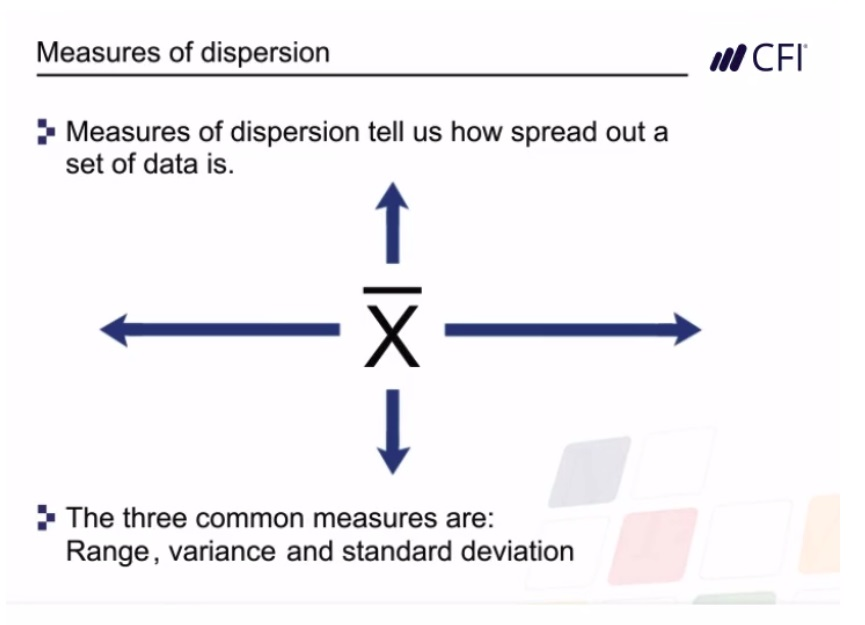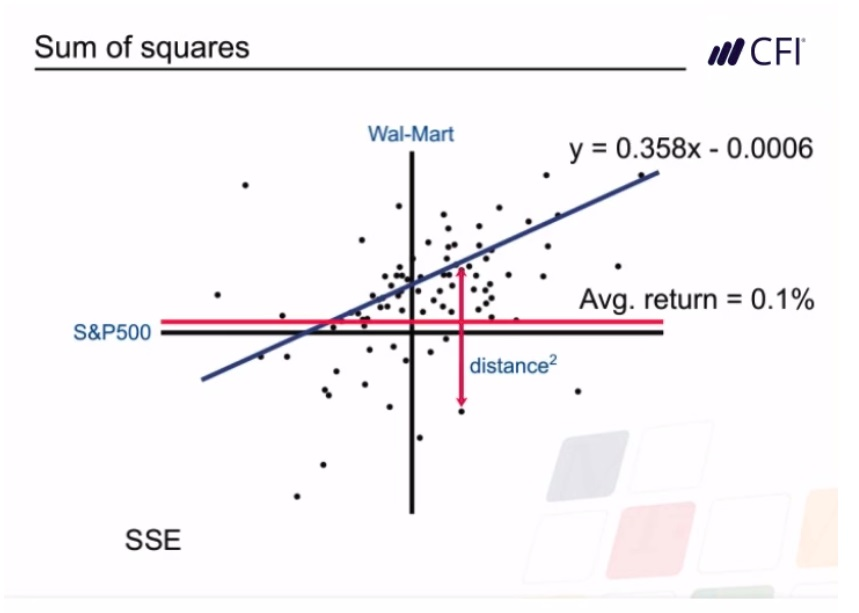## Math for Corporate Finance

Beginner level

Approx 2.50h to complete

100% online and self-paced

## Overview

### Financial mathematics course overview

This four module course demonstrates how financial mathematics formulas can be used to conduct detailed analysis on a set of data and variables.

### Discounted cash flows

In this first module, we explore the concept of “time value of money”. The module focuses on how to calculate present values and future values using compounding and discounting techniques. Additionally, this module goes on to outline how the present values of annuities, perpetuities and growing perpetuities can be calculated. Each concept is reinforced with practical and applied exercises and case studies. By the end of this module, you will have a solid understanding of how discounted cash flow techniques are used to evaluate future cash flows.

• Understand the concept of the time value of money
• Explain terms such as present value, future value, NPV, DCF, annuities, and perpetuities
• Use DCF techniques to calculate present values
• Calculate the present value of cash flow streams such as annuities and perpetuities

### Bond pricing and yields

In this financial mathematics module, we explore bond pricing and yields. The module begins by explaining how to calculate the price of a bond using discounted cash flows. Then, the module goes on to outline how various yields (such as the current yield and the yield to maturity) are calculated. This module also explains the relationship between bond prices and yields as well as how to identify whether a bond is trading at par, at a premium, or at a discount.

Each concept in this module is reinforced with practical and applied exercises and case studies. By the end of this module, you will have a solid understanding of how bonds are priced and yields are calculated.

• Use DCF to find the price of an annual or semi-annual bond
• Calculate the different yields used to assess bond returns
• Understand the relationship between bond prices and yields
• Identify whether a bond is trading at par, premium, or discount### Statistics & financial mathematics

In this module, we explore key statistical measures. The module begins by explaining how to calculate measures of central tendency including the arithmetic mean and expected values. The module then goes on to outline how simple and weighted moving averages are calculated. This module also explains how to calculate different types of statistical dispersion such as range, variance, and standard deviation.

Each concept in this module is reinforced with practical and applied exercises and case studies. By the end of this module, you will have a solid understanding of how to calculate key statistical measures.

• Calculate measures of central tendency including arithmetic mean, weighted mean, WACC, and expected values
• Calculate simple moving and weighted moving averages
• Calculate different types of statistical dispersions such as range, variance, and standard deviation### Covariance, correlation, and regressions

In this financial mathematics module, we examine the mathematical relationship between two variables. The module begins by explaining how covariance and correlation are defined and calculated. The module then moves on to explain how regression analysis is used to estimate the value of one variable based on another. It also explains the regression equation, outlines how to interpret the coefficient of determination, and explains how to access the regression tools in Excel.

Each concept in this module is reinforced with practical and applied exercises and case studies. By the end of this module, you will have a solid understanding of how to calculate and interpret covariance, correlation, and the results of regression analysis.

• Use correlation and covariance to describe the relationship between two variables
• Calculate covariance and correlation using Excel
• Use regression analysis to describe the relationship between two variables
• Undertake regression analysis in Excel and interpret the results### Who should take this financial mathematics course?

This course is specifically designed for finance professionals working in investment banking, equity research, corporate development, investment management, finance, and accounting.

## What You'll Learn

Lesson
Multimedia
Exams
Files
Introduction
Discounted Cash Flows
Bond Pricing and Yields
Key Statistical Skills
Covariance, Correlation and Regression
Qualified Assessment

## Become a certified Financial Modeling & Valuation Analyst (FMVA)®

Math for Corporate Finance is part of the Financial Modeling & Valuation Analyst (FMVA)® certification, which includes 30 courses.

Financial Modeling & Valuation Analyst (FMVA)® Certification
• Skills Learned Financial Modeling and Valuation, Sensitivity Analysis, Strategy
• Career Prep Investment Banking and Equity Research, FP&A, Corporate Development

## Path To Certification

### Prerequisite Courses

7 courses from beginner to intermediate level.

01Optional

### Core Courses

11 courses from beginner to intermediate level.

02Required

### Elective Courses

Take 3 out of 11 courses at an advanced level.

03Required

### Get Certified04Required05Optional

## How the Certification Works

FMVA® Financial Analyst Certification courses are offered 100% online, allowing you the flexibility to start the financial analyst program anytime and learn when it’s most convenient for you.

The program is a part of the All Access Subscription, which includes all programs and courses.

CFI courses are designed by instructors with over 21 years of financial analyst training experience.

Students must complete all core and elective courses (14 in total) and demonstrate mastery of the topics through successful completion of course materials, quizzes, and assessments.
Within 48 hours of program completion, your Blockchain Verified FMVA Certification will be emailed to you.

## What Our Students Say

Math for Corporate Finance
Thank you so much for this course. It clarified so much for me. I feel I have a better grasp of these topics. You guys are the best.

#### Lenny Stanley Cedano

That’s w very good course and an interesting one

#### gasim nasser

Awesome Experience
This Topic was interesting where as a Finance Student it helps in my research paper to draw my assumptions on share and stock price of different companies using statistical tool in excel such as regression, coffecient co-variance analysis and present my data with the help of scattered chart with trend line slopes. which helps the users of my report to understand it easily with any further explaination on my thesis.

#### Janani K.P

Amazing course!
I enjoyed every lessons. The exercises were very helpful.